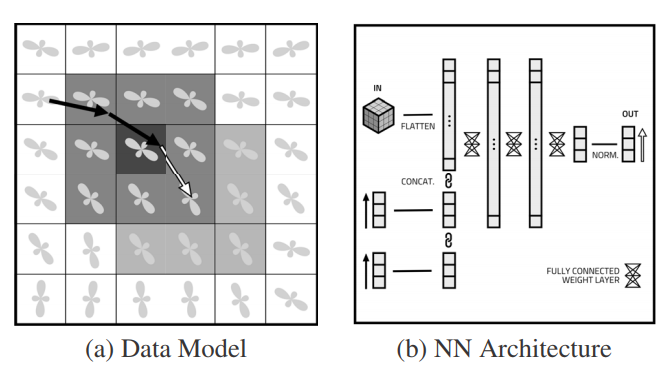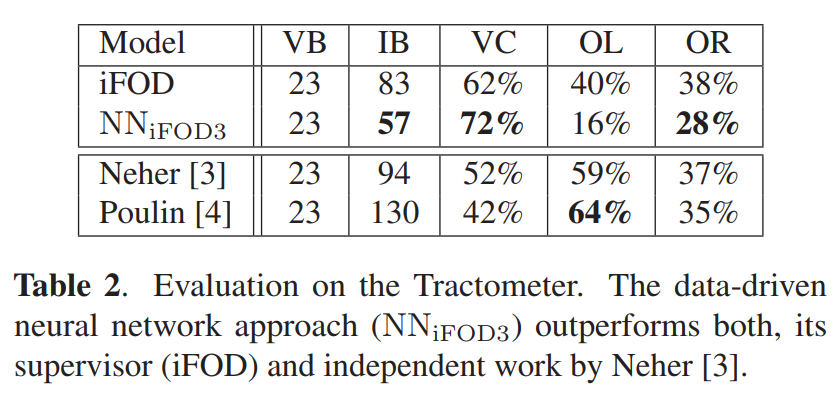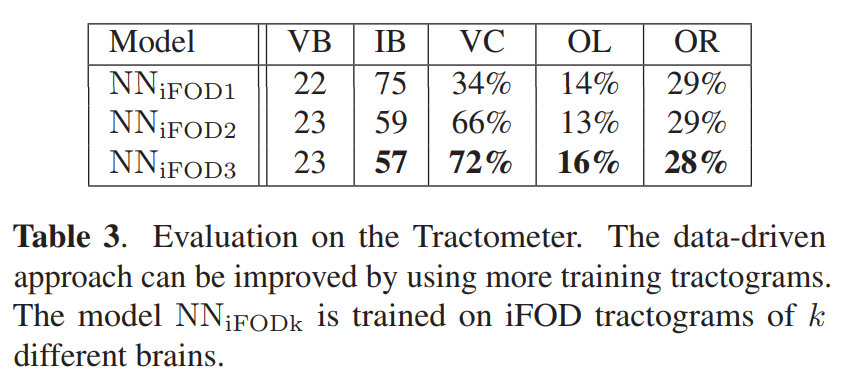## Summary

The main idea is to use a standard feed-forward neural network to predict tracting directions using a context window.### Input

The diffusion signal is first processed using spherical deconvolution to extract fiber ODFs, with order 4 spherical harmonics (15 coefficients).

### Model

The neural network takes as input a 3x3x3 cube around the point of interest, and the last 4 directions as unit vectors. The network is composed of three hidden layers of 2500 units each, then 3 output units and a normalization operation to predict the 3D output direction as a unit vector. The network is trained using a cosine distance loss.

### Datasets

MRtrix probabilistic whole-brain tracking is used on 3 HCP subjects to generate the training data. The 2015 ISMRM tractometer challenge is used to evaluate the model.

### Tracking

Seeding points are placed in the white/grey matter interface, and a step size of $$0.1 \times \text{voxel size} = 0.125\text{mm}$$ is used. The tracking process stops when streamlines hit the white/grey matter boundary again.

## Experiments and Results